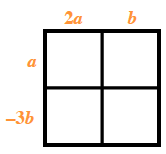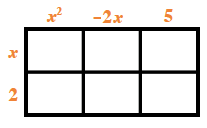Home > CC4 > Chapter 11 > Lesson 11.2.1 > Problem11-55

11-55.
1. Multiply each pair of polynomials. Homework Help ✎

2.  a. (2a + b)(a − 3b) b. (x + 2)(x2 − 2x + 5)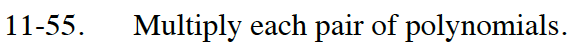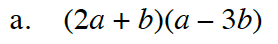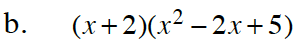Use a generic rectangle to multiply the polynomials.